# Merging Arrays in C

## Merging Arrays in C Language with Examples:

In this article, we will see how we can Merging Arrays in C Language with Examples. In our previous article, we have seen insertion in the sorted array, checking if the given array is sorted or not.

##### Merging Arrays:

Merging is a binary operation. It needs more than one array so that it can combine into a single array. Likewise merging, there are some other binary operations also which we can perform on the array. Those are:

1. Append – it will add elements of another array to the end of the given array.
2. Concat – combining two arrays is concatenation. It creates a 3rd array and then combines the previous two arrays in the 3rd array.
3. Compare – it will check whether two arrays have the same element or not.
4. Copy – if we have 2 arrays and we want to copy all the elements from these two arrays to a 3rd array.

But in this article, we will discuss only merging. Merging can be done only in sorted arrays.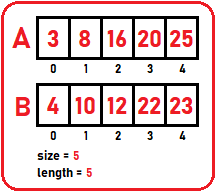The above 2 arrays are already sorted and we have to combine these two arrays and get a single sorted array and for merging, we need definitely 3rd array. We cannot combine and store only in A or in B. we need one more array, let us say C: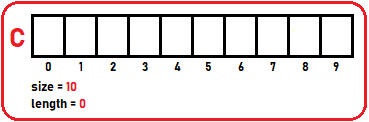Now, before looking at the procedure, let us see the number of elements in array A are m, and the number of elements in the array B is n: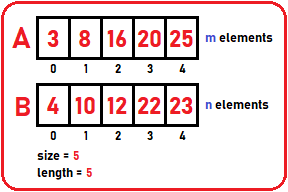Now we will combine these two and store them in the C array such that they are sorted. For storing elements in the C array, we will take index pointers i, j, and k. i start from the 0th index of A, j start from the 0th index of B and k start from the 0th index of C: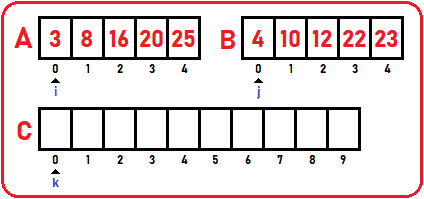Now, the procedure we perform is the repeating procedure. Here we will compare A of i to B of j. whichever is smaller, copy that element to C of k. if A of i is smaller copy it to C or if B of j is smaller copy it to C. Here A of i is smaller. So copy 3 to C  and increment i and k: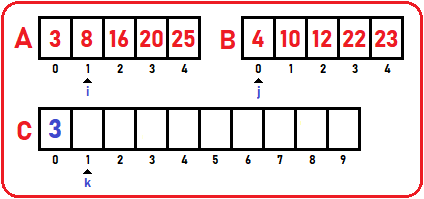Again, compare A of i to B of j. here, B of j is smaller, so copy 4 to C  and increment j and k.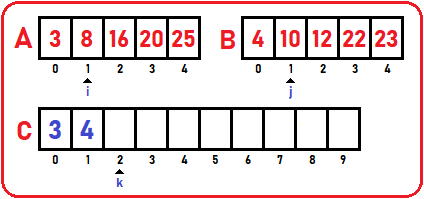Compare A of i to B of j. here, A of i is smaller, so copy 8 to C  and increment i and k.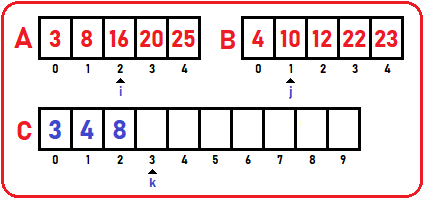Compare A of i to B of j. here, B of j is smaller, so copy 10 to C  and increment j and k.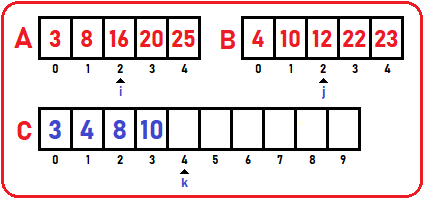Compare A of i to B of j. here, B of j is smaller, so copy 12 to C  and increment j and k.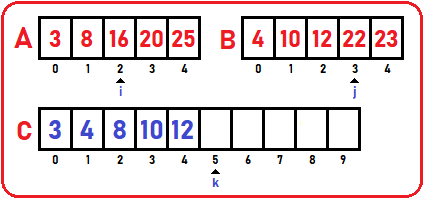In the same manner, elements of both the arrays will compare with each other and copy to C array. Finally, we will get our desire array: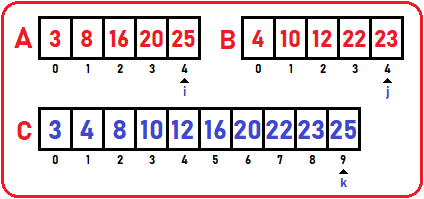If the index pointer value is more than the array’s last index then it means that list will finish. But another list has a few elements left. So, what will we do, when any pointer index goes out of index then we will copy all rest of the elements of another list to array C.? So, we have to end the loop when any of the list’s index pointer will equal to n or m. So, this is the merging process. Let’s have a look at the code:

##### Merging Two Arrays Code in C Language:
```struct List* Merge(struct List *list1, struct List *list2){
int i,j,k;
i = j = k = 0;

struct List *list3=(struct List *)malloc(sizeof(struct List));

while(i < list1->length && j < list2->length){
if(list1->B[i] < list2->B[j])
list3->B[k++] = list1->B[i++];
else
list3->B[k++] = list2->B[j++];
}
for(; i < list1->length; i++)
list3->B[k++] = list1->B[i];
for(; j < list2->length; j++)
list3->B[k++] = list2->B[j];
list3->length = list1->length + list2->length;
list3->size = 10;

return list3;
}
```
##### Analysis:

What is the work done? Comparing the elements and copying the elements. Here we prefer copying the elements. So how many elements are copied? m elements are present in A and n elements are present on B so,

Time Complexity: θ (m + n)

Here we used 2 variables in time complexity. This is the notation of merging.

##### Full Merging Array Code in C language:
```#include<stdio.h>
#include<stdlib.h>

struct List{
int B;
int size;
int length;
};

void Display(struct List list){
int i;
printf("\nElements are\n");
for(i=0;i<list.length;i++)
printf("%d ",list.B[i]);
}

struct List* Merge(struct List *list1, struct List *list2){
int i,j,k;
i = j = k = 0;

struct List *list3=(struct List *)malloc(sizeof(struct List));

while(i < list1->length && j < list2->length){
if(list1->B[i] < list2->B[j])
list3->B[k++] = list1->B[i++];
else
list3->B[k++] = list2->B[j++];
}
for(; i < list1->length; i++)
list3->B[k++] = list1->B[i];
for(; j < list2->length; j++)
list3->B[k++] = list2->B[j];
list3->length = list1->length + list2->length;
list3->size = 10;

return list3;
}

int main(){
struct List list1 = {{3, 8,16,20,25},10,5};
struct List list2 = {{4,10,12,22,23},10,5};
struct List *list3;

printf("First Array:");
Display(list1);

printf("\n\n");

printf("Second Array:");
Display(list2);

printf("\n\nArray after Merging:");
list3 = Merge(&list1,&list2);
Display(*list3);

return 0;
}
```
###### Output: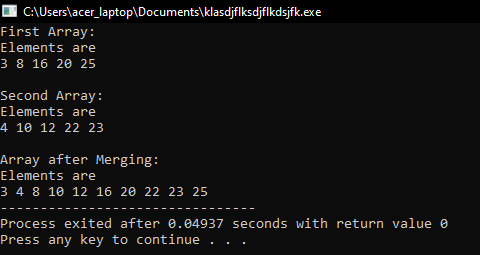In the next article, I am going to discuss Array Set Operations in C Language with Examples. Here, in this article, I try to explain Merging Arrays in C Language with Examples and I hope you enjoy this Merging Arrays in C Language with Examples article.